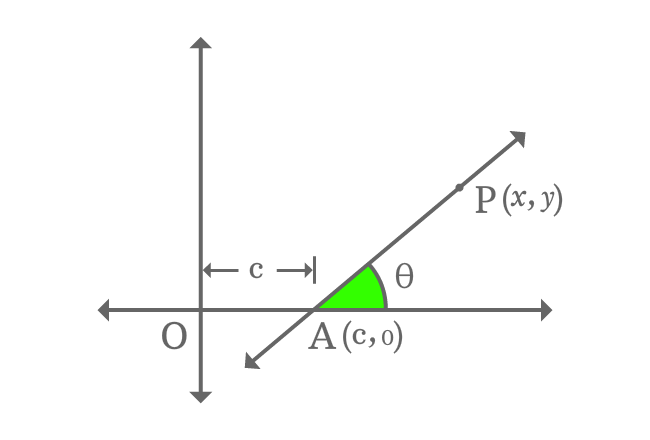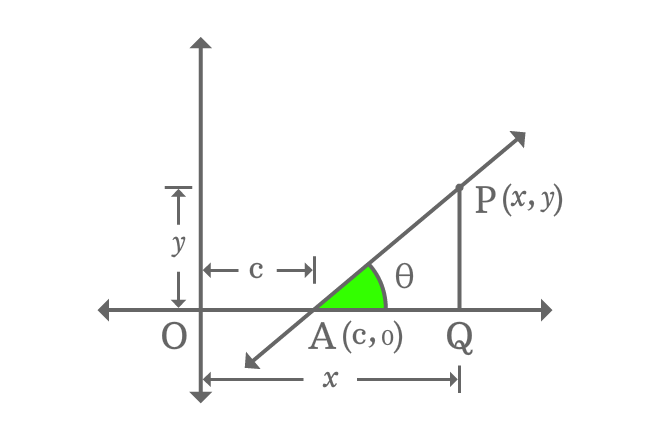# Slope and $\large x$ intercept form of a Line

## Equation

$x \,=\, m’y+c$

It is an equation of a straight line when a straight line intercepts $x$-axis at a point with some slope.

### Proof

A straight line intercepts the horizontal $x$-axis at point $A$ with an intercept $c$ and it makes an angle of $\theta$ with horizontal axis. The point $A$ in the form of coordinates is $A(c, 0)$.$P$ is any point on the straight line and it is $x$ and $y$ units distance from origin in horizontal and vertical axes directions respectively. So, the point $P$ in the form of coordinates is $P(x, y)$.

Therefore, the straight line is represented as $\small \overleftrightarrow{AP}$ geometrically in mathematics.

Assume, the slope of straight line is denoted by $m$ and express it in trigonometric form.

$m \,=\, \tan{\theta}$

Now, draw a perpendicular line to horizontal $x$-axis from point $P$ and it intersects the $x$-axis at point $Q$. Thus, a right triangle ($\Delta QAP$) is formed geometrically.Calculate the $\tan{\theta}$ in trigonometric system and it is equal to the slope of the straight line.

$\tan{\theta} \,=\, \dfrac{PQ}{AQ}$

$\implies \tan{\theta} \,=\, \dfrac{PQ}{OQ-OA}$

$\implies \tan{\theta} \,=\, \dfrac{y}{x-c}$

$\implies m \,=\, \dfrac{y}{x-c}$

$\implies x-c \,=\, \dfrac{y}{m}$

$\implies x \,=\, \dfrac{y}{m}+c$

$\,\,\, \therefore \,\,\,\,\,\, x \,=\, \Big(\dfrac{1}{m}\Big)y+c$

It is a linear equation which represents an equation of a straight line when a straight line intercepts $x$-axis with an $x$-intercept and slope. Hence, it is called slope and $x$-intercept form equation of a straight line.

Take the reciprocal of $m$ as $m’$.

$\,\,\, \therefore \,\,\,\,\,\, x \,=\, m’y+c$

Latest Math Topics
Jun 26, 2023
Jun 23, 2023

###### Math Questions

The math problems with solutions to learn how to solve a problem.

Learn solutions

Practice now

###### Math Videos

The math videos tutorials with visual graphics to learn every concept.

Watch now

###### Subscribe us

Get the latest math updates from the Math Doubts by subscribing us.